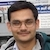# NAEST 2018 Screening Test: BuoyancyBy

A ball is completely immersed in a partially filled glass of water. A string is used to hold the ball at two different positions. In case (A), the ball is at a depth h1 and in case (B), the ball is at a more depth h2 (h2> h1).

Question: As the ball goes from position A to B,

1. The pressure at each point of the ball has increased.
2. The force by the water on the ball has increased.
3. The force by the string on the ball has increased.
4. The level of water in the cylinder has increased.

Solution: Let the depth of the centre of the ball be $h_1$ in case (A) and it is $h_2$ in case (B). Thus, the depth of each point of the ball increases by $h=h_2-h_1$ when it moves from A to B. Thus, hydrostatic pressure at each of the ball increase by \begin{align} p_B-p_A=\rho g h=\rho g (h_2-h_1) \end{align}

The force on the ball by the water is equal to buoyant force ($F_b$) on it. By Archimedes principle, the buoyant force on the ball is equal to the weight of displaced water. This remains the same as the ball moves from A to B.

The force by the string on the ball balances the difference between the ball's weight (W) and the buoyant force ($F_b$) on it i.e., $T=W-F_b$. The forces $W$ and $F_b$ remains constant as the ball moves from A to B. Thus, the tension in the string also remains constant.

The ball is completely immersed in both cases. Thus, the water level in the glass remains the same as ball moves from A to B.

See a detailed explanation of this question by Dr HC Verma.